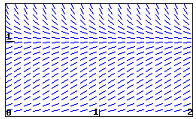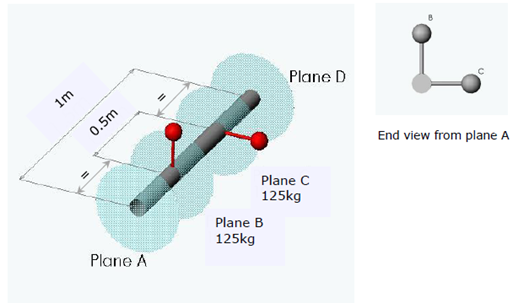+1-415-670-9189
info@expertsmind.com

# Get Solution

Determine the angular velocity of link AB about point B
Course:- Mechanical Engineering
Reference No.:- EM132308143

 TweetExpertsmind Rated 4.9 / 5 based on 47215 reviews.
Review Site
Assignment Help >> Mechanical Engineering

Task 1 - The slider-crank mechanism shown below has a crank radius which is 125mm the mechanism rotates at a speed of 500RPM.

Use a vector diagram to determine the velocity of the piston at the position given in the diagram. Determine the angular velocity of link AB about point B.Task 2 - A two-cylinder engine has a stroke length of 0.3m. The cranks are set at right angles and the mass, which may be considered to be rotating at each crank pin, is 125kg. The planes of rotation of the cranks are 0.5m apart and they are symmetrically placed with respect to two flywheels that are 1m apart.

Use the table below and a vector diagram to find the magnitude and angular positions of the balance masses required in the flywheels at a radius of 0.4m.Task 3 - (Only b) A flywheel has a moment of inertia of 20 kg/m2 and rotator at a speed of 25 rads/s. This is coupled with a second flywheel which is initially rotating at 35 rads/s and has a moment of inertia of 15 kg/m2.

(a) Determine the rotational speed when the clutch plates are engaged.

(b) Calculate the energy lost during engagement.

Minimize

Ask Question & Get Answers from Experts
Browse some more (Mechanical Engineering) Materials
 Write a computer program that, for an area with known moments and product of inertia Ix, Iy, and Ixy, can be used t obtained by rotating the original axes counterclockwise t a cylinder of diameter 200mm and another of diameter 300mm are placed in a channel. If cylinder 2weights 800,1200N determine the reaction developed at contact point anf assu Define prying action? Sketch an alternative joint diagram showing the effects of prying action Name at least two things the joint designer can do to reduce prying action? A radiator wall measuring 2 m by 3 m (WxH) is held a temperature of 125 °C. Straight aluminium fins (k = 175 W/mK) are to be mounted on the wall. The fins are 5 cm in length a Save Your Professor's Life EGE 2013, Prof. J. Kerns Spring 2016. At this point, you have determined the specifications for load factors, bolt strengths, and the strength of t Large stationary brayton cycly gas turbine power plant delivers a powr output of 100MW to an electric generator . Minimum temperature is 300K so T1= 300k max temp is 1600k A simply-supported beam of span 2 m is subjected to a lateral uniformly distributed load of 20 kN/m which causes a maximum vertical shear force of 20 kN at each support. Det A copper wire of diameter D = 200 um and a total length 2L = 200 mm is stretched between two large substrates, each of which is at temperature Tb = 100 C. Air at temperature T